# Numbers Worksheets

Numbers Worksheets for preschoolers to practice reading, writing, and tracing numbers. They will enjoy coloring the objects on each of these worksheets. Simply download and print.

Showing 1-11 of 11 records

•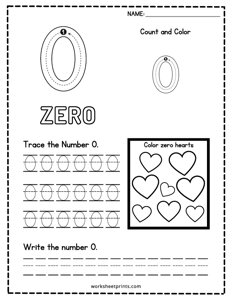Number 0 Practice

sheets, sheet, numbers

•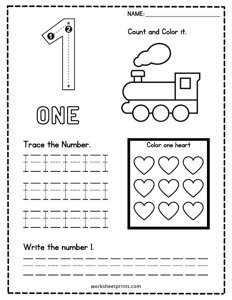Number 1 Practice

sheets, sheet, numbers

•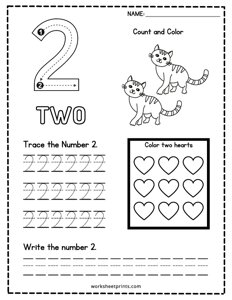Number 2 Practice

sheets, sheet, numbers

•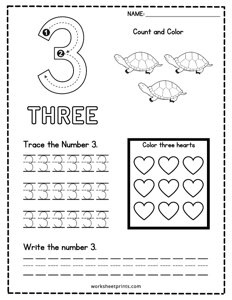Number 3 Practice

sheets, sheet, numbers

•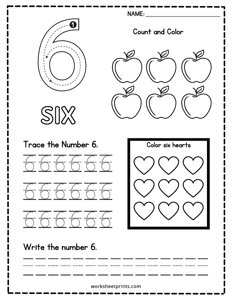Number 6 Practice

sheets, sheet, numbers

•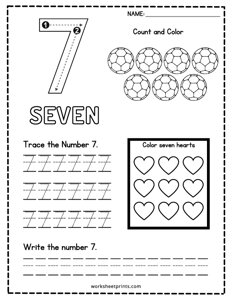Number 7 Practice

sheets, sheet, numbers

•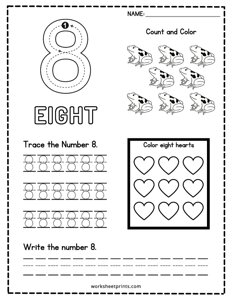Number 8 Practice

sheets, sheet, numbers

•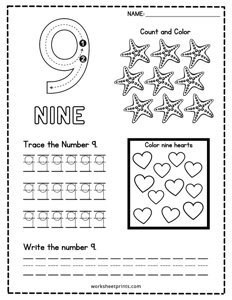Number 9 Practice

sheets, sheet, numbers

•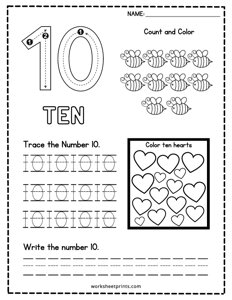Number 10 Practice

sheets, sheet, numbers

•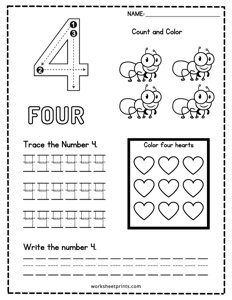Number 4 Practice

sheets, sheet, numbers

•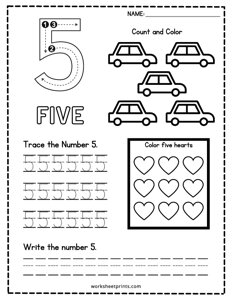Number 5 Practice

sheets, sheet, numbers